## What is persistent homology?

A conceptual introduction to persistent homology and topological data science.

## Ologs

On knowledge representation through ontology logs and how it’s related to category theory.

## Discrete Calculus on Graphs

Text and Mathematica code

Introduction

This is an overview of the discrete differential calculus on graphs with an emphasis on the usage of Mathematica to perform related calculations. While the calculus is more generic and can also be applied to generic simplices, it’s more complex to program since it involves concepts which are not directly deduced from the algebraic properties of graphs (e.g. orientation). We, hence, focus mainly on graphs and the usage of matrix methods.

Much of what is presented here is described in many ways and within various application domains. It’s probably new however to see it presented within Mathematica. This offers tremendous possibilities due to the calculational power and in the ways the various knowledge domains of Mathematica can be combined.

Creating graphs

Mathematica can easily output a wide spectrum of different graph types and offers lots of ways to customize and manipulate graphs. We will use in what follows a collection of graphs to highlight the discrete calculus and the examples below describe the various commands and notations.

Note: each of the gothic symbols L, T, G… can be executed and really are just aliases to Mathematica functions.

Example: a small, random directed graph.

The function R[ ] generates a random Bernoulli graph with five vertices and probability 0.5.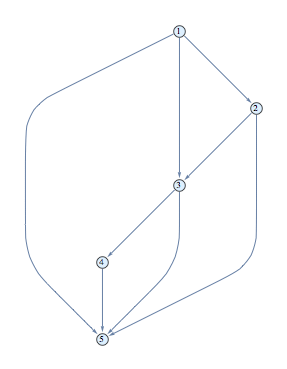You can change the default to produce something larger, e.g. R[12,0.3] produces a random graph with 12 vertices and probability of 0.3 for the edges;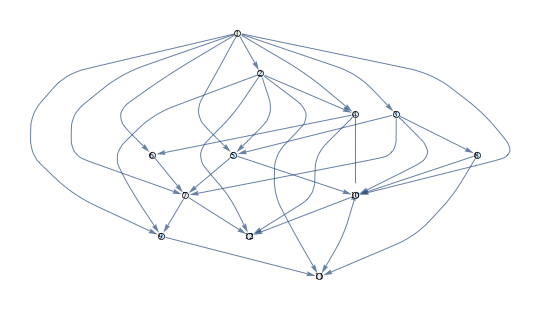Example: the cycle graph.

The gothic O will denote the cycle graph of ten vertices.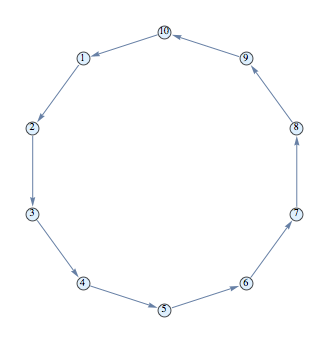Example: the grid graph

The gothic G will denote the grid graph of five colums and three rows.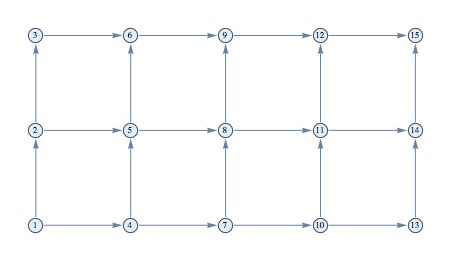Example: the k-ary tree

The gothic T will denote the 3-ary tree of three levels.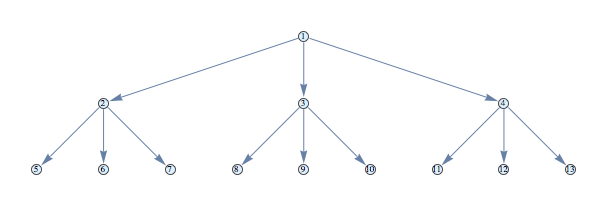Example: the linear graph of ten vertices.

The gothic L will denote the linear chain of ten vertices (9 edges).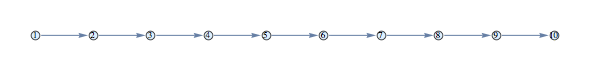Example: the complete graph

The gothic K will denote the complete graph over five vertices.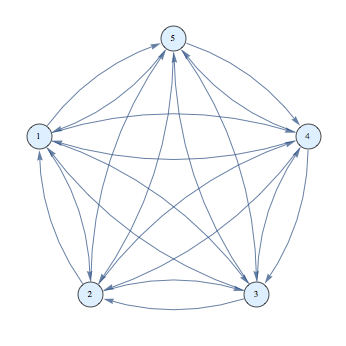Matrices

The notation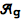or A[g] will denote incidence matrix of the graph g. Note that our incidence matrix is the edge-vertex matrix and not the vertex-edge matrix of Mathematica (i.e. the transposed).

Example: visualizing the incidence matrix

g=R[];
array=A[g];
Row[{g,MatrixForm[array],ArrayPlot[array]}]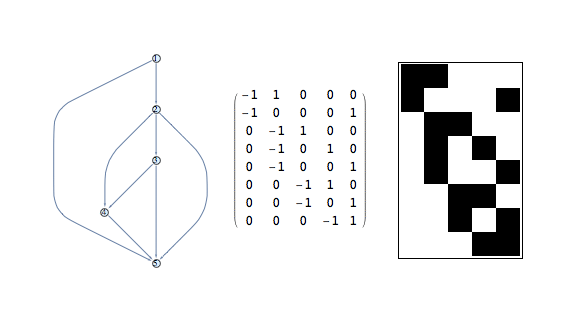Note that the incidence matrix is a sparse array and that if you wish to display it in a standard list you need to convert it using Normal or using MatrixForm for a standard matrix.

It’s important to note that the incidence matrix is not rectangular in general and acts more as a conversion operator from vertices to edges.

The notation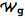or W[g] will denote the adjacency matrix of the graph g. The Mathematica implementation takes the directed edges into account but for our purposes the adjacency matrix needs to be symmetric.

Example: the adjacency matrix of a tree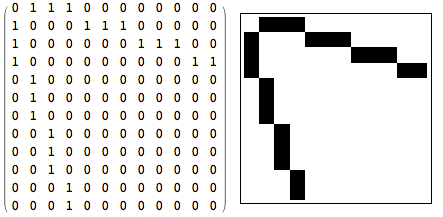The vertex degrees casted in a diagonal matrix will be denoted by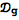or D[g].

Example: the vertex degree matrix of a random graph

ArrayPlot[D[T],ColorRules->{1→Green,0→White,3→Orange, 4→Red}]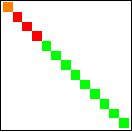The face-edge matrix B representing the incidence of edges with a face depends on the orientation one applies by hand on a face, i.e. a graph or simplex does not have a natural orientation induced by the edges. We assign an orientation to the T, G,… graphs based on the right-hand rule with the thumb point outwards the plane in which the graph resides.

Example: B face-edge matrix

The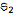graph has one face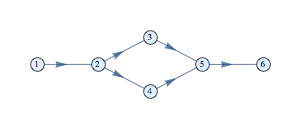and, hence, has a B matrix of one row

B = {{0,-1,1,-1,1,0}}

Note that the order of the B entries corresponds to the order of the edges defining the graph, which in this case is

{12,23, 24,35,45,56}

As a comparison, the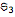is similar but has face edges all pointing in the opposite direction as the face orientation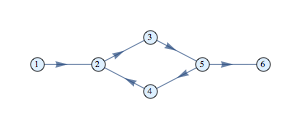B = {{0,-1,-1,-1,-1,0}}

Chains and cochains

A 0-chain is vector defined on the vertices of the graph and 1-chain is a vector defined on the edges of the graph. Note that this is not the same as a function on the graph elements, it’s simply an algebra over the graph elements. A function on the vertices is a 0-cochain and is also a vector but it exists in a different space.

We will use

• σ1, σ2… to denote the 0-chains or vertices
• τ1, τ2… to denote the 1-chains or edges
• τ to denote the whole edge space (the list of all edges)
• σ to denote the whole vertex space (the list of vertices)

For a given graph the makeChainBasis can be used to create the 0 and 1-chain basis elements. One can define higher order elments, like faces (2-chain) and volumes (3-chain) and we will use ocassionally the symbol φ1, φ2… to denote faces.

Example: chains

The 0-chains in the graphare generated (as a vector space) by the six basis elements {σ1, σ2, σ3, σ4, σ5, σ6} and the 1-chains by {τ1, τ2, τ3, τ4, τ5, τ6}.The 0-chain

η = 2 σ1 + 4 σ3 + σ5

corresponds to

{2,0,4,0,1,0}

in the order of the vertices that the graph was defined.

The 1-chain

ζ = 3 τ3 + 7 τ6

corresponds to

{0,0,3,0,0,7}

in the order of the edges that the graph was defined. The linear combination of all vertices σ and the linear combination of all edges τ can be considered as the whole vertex space and edge space respectively.

Dual to the notion of chain are cochains which can be considered as discrete functions on the discrete elements. A 0-cochain is essentially a function on the vertices, a 1-cochain a function on the edges and so on.
We will use
• s1, s2… to denote the 0-cochains or vertex function
• t1, t2… to denote the 1-cochains or edge functions

To generate a symbolic (vertex and/or edge) function on a given graph the makeVertexFunction and makeEdgeFunction can be used.

Example: creating symbolic graph functions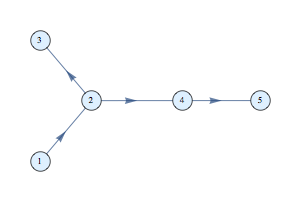A vertex function f has five entries

{f1,f2,f3,f4,f5}

and can be created by means of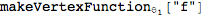Similarly, an edge function has four components

{g12,g23,g24,g45}

create using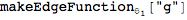Inner product, metric and volume

The inner product defines a pairing between chains and cochains. Conversely, one can look at cochains as duals of the chains. On the zero-th level this means that if η and ζ are chains

< ζ | η > =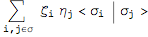R or C

The inner product of the basis elements is defined through a metric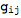=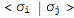.

On the edge level a similar inner product can be defined with a different metric. So, contrary to the continuous case, one can have a hierarchy of metrics and inner products (of different dimensions since the vertex count is not necessarily the same as the edge count).
We will denote by G the metric without specifying the level, the context making clear whether we deal with the metric on a vertex, edge or face level.

The metric can both be seen as a coupling factor in the inner product and as a weight on the vertices and edges. Especially on the edge level it’s interesting that the metric can be taken from the weight of the weighted graph. This means that the metric is usually taken as to be a diagonal matrix with orthogonality; only edge-edge and vertex-vertex coupling are considered.

Much like the continuous calculus you can then go back and forth between chains and cochains of the same level, i.e. for a given edge τ we define the cochain c

c = G τ

and conversely, with a cochain f we associate a chain π

π =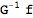This implies that that cochains have an inner product defined as

< f | g > =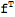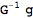Example: metric and weighted graphs

Taking a random graph with random weights assigned to the edges we can form the edge-metric by means of the edgeMetric function, giving for instance the following

g = RandomGraph[{7,7},graphDesign,EdgeWeight→RandomInteger[100,7]]
edgeMetric[g]//MatrixForm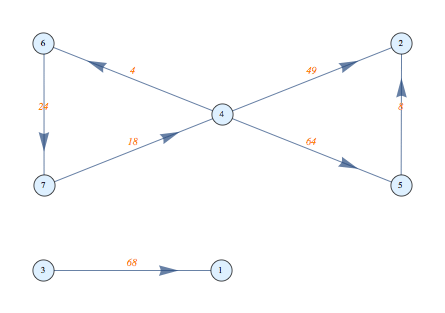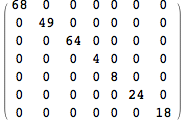The vertex metric can be created similarly with the vertexMetric function.

Because the metric is diagonal and the duality of chains and cochains we can define the inner product of a cochain f with a chain π as follows

< f | π > =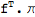which can also be seen as the inner product of two vectors since the chain and cochain spaces are finite.

Finally, the volume of a chain is defined as

Vol = G. 1

which corresponds in the continuous case to the Hodge dual of one.  So, if an integraion of a 1-cochain over an unweighted graph corresponds to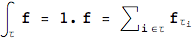while a weighted graphs yields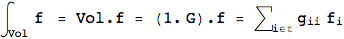Note that if the metric is normalized to Tr(G) = 1 this amounts to the expectation value of f if the metric is seen as a probabilistic distribution.

Example: edge and vertex volume of a random graph

g = RandomGraph[{7,7},graphDesign,VertexWeight→RandomInteger[100,7]];
edgeVolume[g]
vertexVolume[g]

would give something like

{30,42,35,92,16,73,83}
{1,1,1,1,1,1,1}

Integral, boundary and differential operators

We define the integral of a cochain c over a chain η to be equal to the inner product of c and so that the integral of a cochain c over a chain η is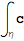= < c | η >

The boundary operator is defined as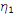=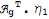for a 1-chain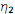=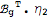for a 1-chainwhich are in fact the only way that the respective matrices can be used to transform one type of vector to another (of a different dimension in general).

Example: the boundary operator

Let g be thegraph and recall that the B matrix for this graph is

{0,-1,1,0,0,0}while the A matrix is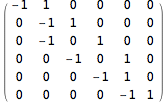Because there is only one face it means that the boundary of this face is the same as B considered as a vector rather than a matrix. This indeed corresponds to the four edges with a sign indicating whether it’s traversed in the same or opposite direction.

Taking a 1-chain η = τ1+τ2+τ4 = {1, 1, 0, 1, 0, 0} the boundary is

∂η = {-1,0,0,0,1,0}

which corresponds to σ5 – σ1 and are indeed the endpoints of the line (sub)graph between vertices 1 and 5.

If we take the integral of a vertex function over the boundary of the full linear chain L we get the difference between the endpoints;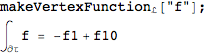This is in essence the fundamental theorem of calculus in the graph context. A consequence of this is that any loop integral is zero. Of course, the general case is not always comparable to the coninuous calculus.

Example: integral over the grid graph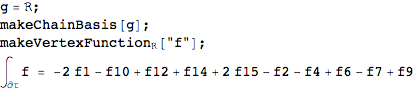Since the cycle graph has no boundary, it’s obvious that the integral always gives zero. The discrete calculus is more general than just graphs and embraces simplices in general. If we would includes 2-chains or higher order elements we could show that in general the boundary of a boundary is zero. Mathematica does not have a simple function to output higher order boundary operators (like the IncidenceMatrix) and it would also require the concept of orientation.

The coboundary (derivative) d of a cochain is defined as the transpose of the boundary operator=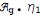for a 1-chain=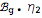for a 1-chainwhich is again the only way one can, in fact, turn a vector from one space into another taking the underlying topology of the graph into account.

From a strict mathematical point of view the coboundary operator would be introduced in analogy to the continuous case:

< ∂σ | f > = < σ | df >

which is obviously true in this case since the respective matrices are transpose of each other.

Example: integral of a differential over L

Taking the linear L this leads to the usual difference

g=L;
makeChainBasis[g];
makeVertexFunction[“f”];
df = {-f1+f2,-f2+f3,-f3+f4,-f4+f5,-f5+f6,-f6+f7,-f7+f8,-f8+f9,f10-f9}

and taking the integral of the linear chain gives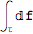= -f1+f10

So, the integral over the linear chain of the differential of the function f is the difference between the endpoints, as should be.

Of course, this is by construction true for any graph and not just the linear chain. So, we have Stoke’s theorem for the discrete calculus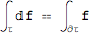for arbitrary graphs. The extension to higher order chains and cochains is easily done theoretically but more difficult to implement in Mathematica, as said earlier, due to the need for orientations on a simplex or chain.

The differential of the 0-chains is not always a basis of the 1-chains, as is the case in the continuous calculus. For example, thegraph has dσ1 + dσ2 + dσ3 = 0.This is also apparent from the fact that the incidence matrix as a rank one eigenspace. It can be easily shown that the egenspace has rank (m-n+1) with m edges, n vertices. So in this case we have effectively a cycle (when disgarding the direction of the edges).

The boundary and coboundary operators are nilpotent as should be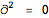and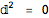corresponding to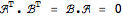.

The gradient of a scalar (0-cochain) u is defined as

∇ u ⇔ A u

which again is the only meaningful way in which one vector space element can be transformed to another. Also note that this corresponds to the differential du which is identical in the continuous context.The divergence of a vector (1-cochain) v is similarly defined as

∇.v ⇔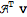Taking the divergence of a gradient leads to the Laplacian and is hence equal to

Δ ⇔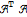Example: diffusion on the triangle graph

The discrete diffusion equation on a graph with discrete time can be written as

u(t+1) = u(t) – α Δu(t)

where α acts as a diffusing coefficient from a vertex to its adjacent vertices. This can be taken as the edge weights or a global constant. If we take for example the following random initial state on the triangle graph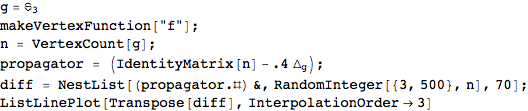we get an equillibrium state after an initial oscillatory transition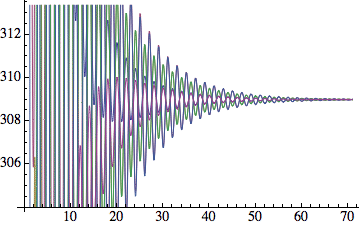but much depends on the initial state and the diffusion coefficient used.

It can be shown that the Laplacian satisfies

Δ = DW

and hence that a stable state means that (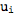=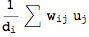meaning that the value at a vertex is the average of the values of its neighbors.

Non-commutative differential algebra

Diifferential structures on discrete sets are never far off from non-commutative algebras and intriguing relationships with stochastics, KdV and other equations. Contrary to the continuous settings one can have many differential structures and topologies on a discrete ‘manifold’.

The vector space of 1-cochains can be turned into a bi-algebra by means of the multiplication ◦ of a 0-cochains f and g as

(f ◦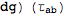=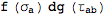=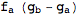(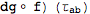=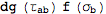=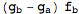which turns it into a non-commutative algebra with commutator

[dg, f]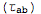=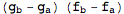so that

∫ [f,df] =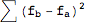which is often referred to as the energy of graph. In a more refined analysis one could proof that when one goes to a continuous limit the commutator vanishes and thus recover the de Rham calculus. Symbolically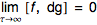Note that the Leibniz property and other differential properties are satisfied

d(fg) = df ◦ g + f ◦ dg = Af . g + f . Ag

due to the fact that the incidence matrix transfers a vertex function to a difference across the edges.

References

Discrete Calculus, J.Grady & J.Polimeni, Springer.

Discrete Exterior Calculus, Hanil Hirani, PhD Thesis.

• Discrete Differential Manifolds and Dynamics on Networks, A.Dimakis, F.Muller-Hoissen & F.Vanderseypen, hep-th/9408114.